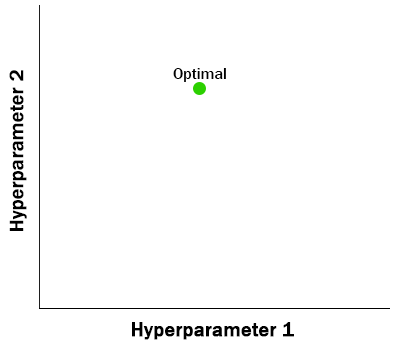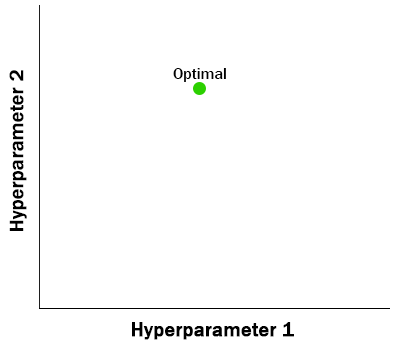## Introduction to Hyperopt

Hyperopt is a popular tool for optimizing hyperparameters in machine learning and data science.## Introduction

Tuning hyperparameters unlocks performance in machine learning models yet can introduce a set of computational challenges. The popular tool hyperopt has emerged as an approach to strike a balance trading off between higher computational demand and model performance. Let’s look at an example of using hyperopt to tune a machine learning model’s hyperparameters - but first we need to understand what are hyperparameters, their challenges, what other approaches exist, and why hyperopt can help.

### Hyperparameters

In machine learning, a hyperparameter is a parameter whose value is used to control the learning process. By contrast, the values of other parameters (typically node weights) are derived via training. - Wikipedia

As the Wikipedia definition above indicates, a hyperparameter controls how the machine learning model trains. Nearly every modern machine learning algorithm affords hyperparameter tuning as there are rarely one-size-fits-all solutions. For example, in a random forest you may want to specify the maximum depth (number of levels a single decision tree can reach), the number of estimators (the number of trees in the forest), the maximum features (considered at each split), and so on. When training a neural network, the hyperparameters you may care about include learning rate (how quickly parameters are updated), the number of epochs (how often training data is provided to the neural network), and the number of hidden layers (how deep is your network). In both examples, the hyperparameters are not what are learned but what guide the learning process.

As you may begin to imagine, the number of combinations of hyperparameters can grow rapidly as you wish to tune more hyperparameters, consider more options for a given hyperparameter, or both. There now exists a search space of hyperparameter values - but determining the best combination is a non-trivial task.

Before diving in to the workings of hyperopt, let’s take a look at the motivation using alternative search methods. The two most common approaches to searching for optimal hyperparameters are grid search and random search - each with advantages and disadvantages.

Grid Search

Grid search relies on a parameter grid defined upfront to specify every value of interest for every hyperparameter to be evaluated. All evaluations have been pre-determined.

Random Search

Random search relies on drawing random values from distributions defined for each hyperparameter. Each evaluation is independent of previous evaluations.

Grid Search• Simple to parallelize
• Suffers from the curse of dimensionality
• Not guaranteed to find optimal solution
• Nothing learned in search t from search t-1
Random Search• Simple to parallelize
• Suffers from high variance
• May return better results than grid search
• Nothing learned in search t from search t-1

While both approaches are often superior to manual hyperparameter tuning, neither grid search nor random search make use of the previous evaluations. In the next section we look at an approach that does consider past performance.

### Tree-Structured Parzen Estimator (TPE)

The Tree-structured Parzen Estimator approach is a sequential model-based optimization (SMBO) algorithm that finds the inputs to an objective function that maximizes expected improvement, EI. The expected improvement depends on a threshold parameter, γ (gamma) which can be adaptive, the distribution of previous evaluations, 𝓁(x), better than the current gamma threshold, and the distribution of previous evaluations, g(x), worse than the current gamma threshold. The following expression, taken from the Bergstra paper linked below, captures the relationship between these inputs.

$$EI_{y*}(x) \propto (\gamma + {g(x)\over \ell(x)} (1 - \gamma))^{-1}$$

For more information and a deeper explanation, read the source paper here: Algorithms for Hyper-Parameter Optimization by Bergstra et al. The critical takeaway is that the algorithm uses previous evaluation results to determine the best next search option or set of hyperparameters.

## Installation

Installation of hyperopt is simple and can be completed in most cases using a single command like the ones below. Once installed, there isn’t much if any configuration that you’ll need to complete - we can pass most parameters directly to hyperopt functions.

From PyPI
# From PyPI
pip install hyperopt

From Conda-forge
# From Conda-forge
conda install -c conda-forge hyperopt

With hyperopt now installed we can begin with the example optimization. Let’s walk through a basic example with a simple objective function and search space.

## Example: Using Hyperopt

For this example of using hyperopt we will optimize the hyperparameters for a random forest classifier. At its simplest we need to complete four steps to use hyperopt:

1. Define the objective function
2. Describe the search space
3. Optimize the objective function (i.e., call fmin())
4. Analyze the results

### Basic example

In this basic example we train a random forest classifier and optimize these hyperparameters with hyperopt: criterion, n_estimators, max_depth, and max_features. As this is merely a demonstration of hyperopt, we are optimizing accuracy but you could optimize for any other scalar-valued loss function.

 1from sklearn.ensemble import RandomForestClassifier
2from sklearn.model_selection import train_test_split
3from sklearn.metrics import accuracy_score
4from hyperopt import hp, fmin, tpe, Trials, STATUS_OK
5from hyperopt.pyll import scope
6
7# Perform train test split
8X_train, X_test, y_train, y_test = train_test_split(X, y, test_size=0.20, random_state=2021)
9
10# Define objective function
11def objective(params, X, y):
12    # Define classifier
13    random_forest = RandomForestClassifier(**params,n_jobs=-1)
14
15    # Fit random forest
16    random_forest.fit(X_train, y_train)
17
18    # Predict on test
19    y_pred = random_forest.predict(X_test)
20
21    # Get accuracy
22    accuracy = accuracy_score(y_test, y_pred)
23
24    # Return results
25    return {"loss": -1 * accuracy,
26            "status": STATUS_OK,
27            "eval_time": time.time()
28           }
29
30# Define parameter space using hyperopt random variables
31param_space = {
32    "criterion": hp.choice("criterion", ['gini', 'entropy']),
33    "n_estimators": scope.int(hp.quniform("n_estimators", 10, 100, 10)),
34    "max_depth": scope.int(hp.quniform("max_depth", 2, 8, 1)),
35    "max_features": hp.choice("max_features", ['sqrt', 10, 15])
36}
37
38# Set up trials for tracking
39trials = Trials()
40
41# Pass objective fn and params to fmin() to get results
42results = fmin(
43    objective,
44    space=param_space,
45    algo=tpe.suggest,
46    trials=trials,
47    max_evals=150
48)

Once this code completes execution, you can explore the results object to see the best found hyperparameters. While this approach may be faster to converge on an optimal solution than doing grid search or random search, if your data set is sufficiently large, you will need to use distributed computing to both train and tune your model.

### Running with SparkTrials

Using hyperopt on Spark is simple as only a few lines of code need to be swapped. Look at the code snippet below to see how to change the imported Trials object and define parallelism to be used.

Before proceeding, consulting this handy guide from Databricks which explains the best practices using hyperopt in a distributed compute environment: Databricks - Hyperopt Best Practices. Examples include avoiding the use of SparkTrials if autoscaling is enabled and understanding if you are in a CPU or GPU cluster.

 1# Swap out Trials object for SparkTrials
2from hyperopt import SparkTrials
3
4...
5
6# Using the SparkTrials object you can define the parallelism
7spark_trials = SparkTrials(parallelism = 24)
8
9# Now pass the spark_trials object to fmin()
10results = fmin(
11    objective,
12    space=param_space,
13    algo=tpe.suggest,
14    trials=spark_trials,
15    max_evals=150
16)

## Conclusion

From both a conceptual description and practical example, we can see that hyperparameter tuning can be performed better and faster using automated tools like hyperopt. Other tools exist (e.g., Optuna) that perform similar functions and, depending on your use case, may be more beneficial to use.

### Bayesian Optimization

If you are interested in hyperparameter tuning and want to learn more, you should check out some of the resources below and look into Bayesian optimization (of hyperparameters). A great example is the Bayesian optimization framework BoTorch which pairs nicely with ax. The paper for BoTorch can be found here.